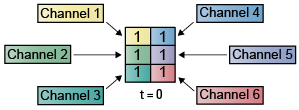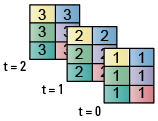## Create Multichannel Signals for Sample-Based Processing

In sample-based processing, blocks process signals one sample at a time. Each element of the input signal represents one sample in a distinct channel. For example, from a sample-based processing perspective, the following 3-by-2 matrix contains the first sample in each of six independent channels.When you configure a block to perform sample-based processing, the block interprets scalar input as a single-channel signal. Similarly, the block interprets an M-by-N matrix as multichannel signal with M*N independent channels. For example, in sample-based processing, blocks interpret the following sequence of 3-by-2 matrices as a six-channel signal.For more information about the recent changes to frame-based processing, see the Frame-based processing changes section of the DSP System Toolbox™ Release Notes.

### Multichannel Signals for Sample-Based Processing

When you want to perform the same operations on several independent signals, you can group those signals together as a multichannel signal. For example, if you need to filter each of four independent signals using the same direct-form II transpose filter, you can combine the signals into a multichannel signal, and connect the signal to a single Biquad Filter block. The block decides to treat each element of the input as a channel when you set the block's Input processing parameter to ```Elements as channels (sample based)```. The block then applies the filter to each channel independently.

Multiple independent signals can be combined into a single multichannel signal using the Concatenate (Simulink) block. In addition, several multichannel signals can be combined into a single multichannel signal using the same technique.

### Create Multichannel Signals by Combining Single-Channel Signals

You can combine individual signals into a multichannel signal by using the Matrix Concatenate (Simulink) block in the Simulink® Math Operations library:

1. Open the Matrix Concatenate Example 1 model by typing

`ex_cmbsnglchsbsigs `

at the MATLAB® command line.2. Double-click the Signal From Workspace block, and set the Signal parameter to `1:10`. Click .

3. Double-click the Signal From Workspace1 block, and set the Signal parameter to `-1:-1:-10`. Click .

4. Double-click the Signal From Workspace2 block, and set the Signal parameter to `zeros(10,1)`. Click .

5. Double-click the Signal From Workspace3 block, and set the Signal parameter to `5*ones(10,1)`. Click .

6. Double-click the Matrix Concatenate block. Set the block parameters as follows, and then click :

• Number of inputs = `4`

• Mode = ```Multidimensional array```

• Concatenate dimension = `1`

7. Double-click the Reshape block. Set the block parameters as follows, and then click :

• Output dimensionality = `Customize`

• Output dimensions = `[2,2]`

8. Run the model. In the Simulation tab, click .

Four independent signals are combined into a 2-by-2 multichannel matrix signal.

Each 4-by-1 output from the Matrix Concatenate block contains one sample from each of the four input signals at the same instant in time. The Reshape block rearranges the samples into a 2-by-2 matrix. Each element of this matrix is a separate channel.

Note that the Reshape block works column wise, so that a column vector input is reshaped as shown below.The 4-by-1 matrix output by the Matrix Concatenate block and the 2-by-2 matrix output by the Reshape block in the above model represent the same four-channel signal. In some cases, one representation of the signal may be more useful than the other.

9. At the MATLAB command line, type `dsp_examples_yout`.

The four-channel signal is displayed as a series of matrices in the MATLAB Command Window. Note that the last matrix contains only zeros. This is because every Signal From Workspace block in this model has its Form output after final data value by parameter set to ```Setting to Zero```.

### Create Multichannel Signals by Combining Multichannel Signals

You can combine existing multichannel signals into larger multichannel signals using the Simulink Matrix Concatenate (Simulink) block:

1. Open the Matrix Concatenate Example 2 model by typing

```ex_cmbmltichsbsigs ```

at the MATLAB command line.2. Double-click the Signal From Workspace block, and set the Signal parameter to `[1:10;-1:-1:-10]'`. Click .

3. Double-click the Signal From Workspace1 block, and set the Signal parameter to `[zeros(10,1) 5*ones(10,1)]`. Click .

4. Double-click the Matrix Concatenate block. Set the block parameters as follows, and then click :

• Number of inputs = `2`

• Mode = ```Multidimensional array```

• Concatenate dimension = `1`

5. Run the model.

The model combines both two-channel signals into a four-channel signal.

Each 2-by-2 output from the Matrix Concatenate block contains both samples from each of the two input signals at the same instant in time. Each element of this matrix is a separate channel.

Watch now